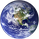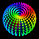# Moving CO-covariance (covariance on covariance)

618 vizualizações
This is Covariance on Covariance. It shows you how much a given covariance period has deviated from it mean over another defined period. Because it is a time series, It can allow you to spot changes in how covariance changes. You can apply trend lines , Fibonacci retracements, etc. This is also volume weighting covariance.

This is not a directional indicator nor is moving covariance. This is used for forecasting volatility . This must be used in conjunction with moving covariance.
//Moving Co-Covariance
study(title="Co-Covariance", shorttitle="MCCV", overlay=false)
src = vwap, len = input(30, minval=1, title="covariance length"), len2 = input(7, minval=1, title="Co-covariance look back length")
mean = vwma(src, len)
stdev = stdev(src, len)
covariance = (stdev/mean)
covariancemean = vwma(covariance, len2)
cstdev = stdev(covariance, len2)
cocovariance = (cstdev/covariancemean)
plot(cocovariance, title="covariance on covariance", style = line, color = aqua)


## ComentáriosI would like to apologize, when naming this indicator I mixed up my terminology. This is coefficent of variation which shows the % a price deviates from its mean. Also known as unitized risk. In this case unitized risk of coefficent of variation
RespostaIn this indicator I forgot to multiply the result by 100. 0.0928 would be 9.28% etc. A small adjustment to the code will alleviate this. Just multiply cocovariance by 100, but if you like to read it as decimal points then this shouldn't matter to you.
Respostainteresting, so based on this info ... we can expect a downward motion soon?
Respostamiquael
or maybe just more very gradual incline up ?
Respostamiquael
This isn't a directional indicator. It is for forecasting volatilty of volatilty. In this sense you can think of it as a 2nd rate derivative of volatility. By this, you should expect volatility to deviate 9.28% this week from the current expected 6.08% deviation over the next 30 days. Likewise the probability of this deviation forecast falls by (1/(Period length - number of days)). From a maximum of 68.26% probability derived from the empirical rule about the percent of data points 1 stdev from their mean. You can also apply trendlines and fib retracements in order to arrive at targets for both vol and vol on vol.
Resposta